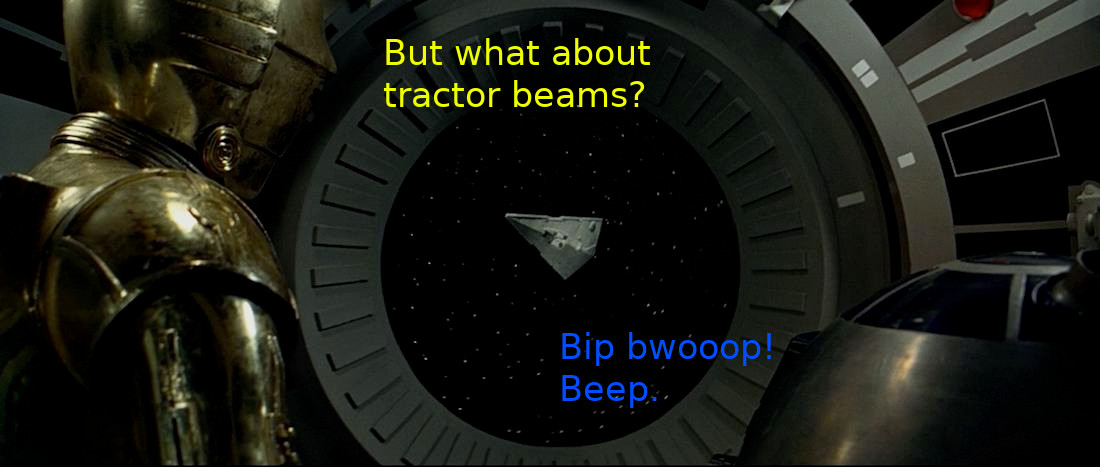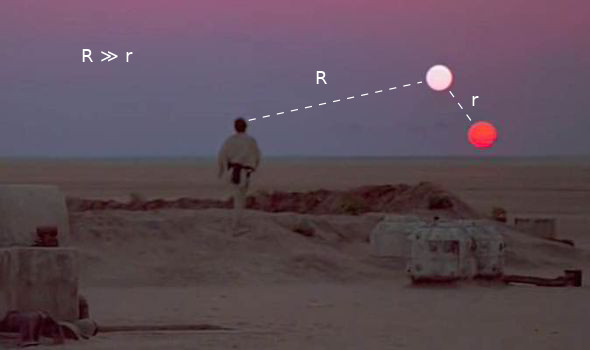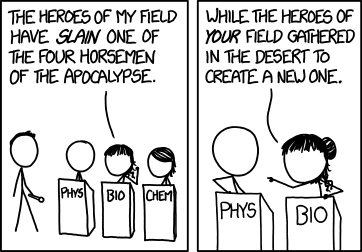# 2

Challenge: Derive general relativity from special relativity via thought experiments.

Consider a spaceship with its windows blacked out. Accelerating at 1 feels the same as sitting on the launchpad. Relativistically, an object resting on the surface of the Earth is accelerating upward at 1 and an object in freefall is at rest along its geodesic. The natural (inertial) path of an object follows its geodesic along the spacetime manifold. Lagrangianly, an object's trajectory locally maximizes proper time.If objects in freefall follow their geodesics then gravity must modify the geodesic itself i.e. gravity warps spacetime.

For gravity to be attractive instead of repulsive, time must be slowed in a gravity well. Under special relativity, slowing time and contracting space are the same thing. Therefore gravity slows time and contracts space. Gravity is produced by mass, so matter emits a field that slows time and contracts space.

# Dyson Sphere over Tatooine

Now consider the gravity potential of a binary star system observed from a distance far, far away compared to the orbital radius between the stars.If it is 1915 and you have not invented general relativity yet then you might believe represents total enclosed rest mass. And it could…if we lived in a Newtonian universe. But we live in a relativistic universe and Gauss' law applies to any closed system.

If you built a Dyson sphere around the binary star then the external gravity of your closed system should never change even as the stars spiral into each other. But as the stars spiral into each other they increase their spatial speed , thereby decreasing their temporal speed . The slower temporal speed (increased Lorentz factor) decreases the stars' gravitational output per unit mass via time dilation. But the total gravity emitted by the closed system (Dyson sphere) must stay constant. The mass of the stars increases to counteract time dilation. Therefore the relativistic mass of a particle equals the rest mass divided by the temporal speed .

The term in Gauss' law is the total relativistic mass plus the energy of the field.

# Gathering in a Desert

You can show the energy density of a field is proportional to the square of the field strength with pure mathematics. No science necessary. By plugging this math into the binary star system throught experiment we find that the energy from the gravitational field gets turned into mass. Thus the total mass-energy is conserved.

What if we did this in reverse? What happens when a Uranium-235 nucleus absorbs a neutron?Just as an observer from outside the Dyson sphere cannot distinguish between the rest mass of the stars from the energy within the enclosed gravitational field, an observer outside a Uranium-235 nucleus cannot distinguish between the mass from the energy enclosed within it. An external observer observes the nucleus's internal energy as mass. So if the rest mass of the products is less than the rest mass of the reactants then the difference is released as energy.

1. Please humor me by ignoring the energy lost to thermal radiation and gravitational waves. ↩︎

New Comment